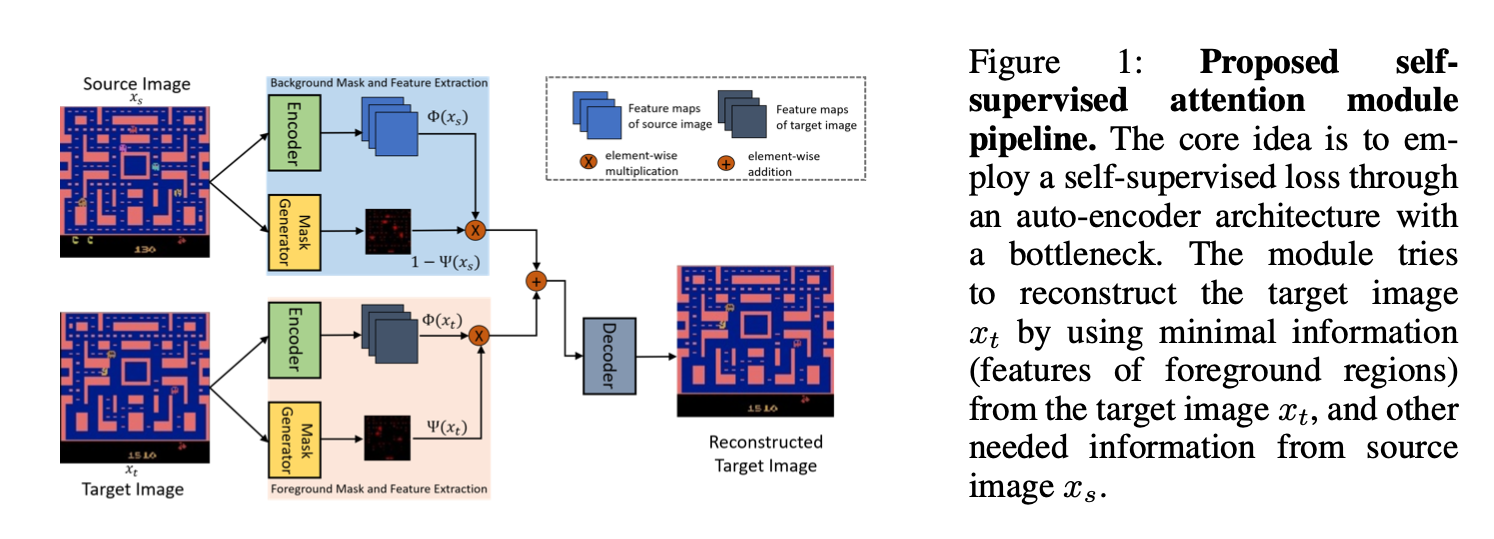# Self-Supervised Attention-Aware Reinforcement Learning¶

link

## Main Idea¶

Self-supervisedly select regions of interst without explicit annotations.The attention is learned with a self-supervised learning loss.
The target image and source image are sampled randomly from a task.
Region of intersts are the fontground, background otherwise.
Note the Mask generator and encoder have shared weights.

The auto encoded feature is $$\hat{\Phi}\left(\boldsymbol{x}_{s}, \boldsymbol{x}_{t}\right) \triangleq\left(1-\Psi\left(\boldsymbol{x}_{s}\right)\right) \cdot\left(1-\Psi\left(\boldsymbol{x}_{t}\right)\right) \cdot \Phi\left(x_{s}\right)+\Psi\left(\boldsymbol{x}_{t}\right) \cdot \Phi\left(x_{t}\right)$$

I am not quite sure why after the plus of outputs of the 2 extractions, it applies the attention $\left(1-\Psi\left(\boldsymbol{x}_{s}\right)\right)$ again.

## Mesurement of the mask generator learned¶

### Single-task learning¶

Train on single task.

### Multi-task learning¶

Train one mask over multi-tasks.

### Transfer mask across tasks¶

Train one mask on one tasks and transfer it to multi-tasks.

### Object Extraction¶

Extact object keypoints with mask learned.